### Bow-ties are CoolHover over the thumbnail for a full-size version.

Author ]{NO3 author:]{no3 n-art nart nonplayable rated 2012-09-21 2012-09-21 5 by 11 people. \$Bow-ties are Cool#]{NO3#nonplayable#00000000000000000000000000000000000000000000000000000000000000000000000000000000000000000000000000000000000000000000000000000000000000000000000000000000000000000000000000000000000000000000000000000000000000000000000000000000000000000000000000000000000000000000000000000000000000000000000000000000000000000000000000000000000000000000000000000000000000000000000000000000000000000000000000000000000000000000000000000000000000000000000000000000000000000000000000000000000000000000000000000000000000000000000000000000000000000000000000000000000000000000000000000000000000000000000000000000000000000000000000000000000000000000000000000000000000000000000000000000000000000000000000000000000000000000000000000000000000000|12^485,169!12^482,172!12^479,176!12^477,180!12^476,185!12^800,605!12^475,190!12^474,195!12^474,201!12^475,206!12^476,212!12^477,217!12^479,223!12^479,228!12^480,234!12^482,239!12^482,245!12^483,250!12^484,255!12^485,260!12^488,266!12^489,272!12^491,277!12^492,283!12^493,288!12^494,290!12^485,263!12^488,274!12^491,281!12^493,287!12^494,293!12^488,166!12^491,161!12^493,158!12^496,154!12^499,151!12^503,146!12^506,143!12^509,140!12^512,137!12^517,131!12^434,352!12^437,348!12^442,347!12^446,350!12^452,353!12^449,349!12^452,352!12^457,354!12^461,356!12^467,356!12^467,355!12^455,353!12^461,353!12^466,355!12^468,354!12^470,356!12^476,355!12^477,354!12^472,355!12^477,353!12^482,351!12^485,349!12^490,347!12^494,344!12^496,340!12^497,338!12^496,295!12^498,301!12^497,302!12^494,295!12^497,301!12^499,305!12^500,311!12^499,316!12^500,321!12^500,327!12^499,332!12^497,337!12^494,341!12^491,344!12^487,348!12^434,353!12^431,356!12^428,361!12^428,366!12^428,371!12^429,377!12^429,383!12^428,388!12^429,393!12^429,399!12^427,403!12^425,407!12^422,410!12^419,413!12^416,415!12^411,417!12^407,418!12^407,418!12^379,426!12^379,432!12^380,437!12^379,441!12^378,446!12^376,450!12^374,455!12^370,458!12^365,460!12^361,461!12^357,465!12^354,468!12^350,472!12^348,476!12^346,479!12^346,484!12^346,488!12^347,494!12^347,500!12^348,505!12^348,508!12^347,513!12^347,518!12^345,522!12^344,524!12^343,529!12^341,534!12^334,542!12^339,538!12^338,538!12^377,419!12^373,416!12^369,414!12^368,414!12^323,410!12^323,409!12^327,406!12^331,404!12^335,402!12^339,401!12^344,399!12^349,398!12^350,397!12^328,410!12^332,413!12^335,416!12^340,418!12^345,419!12^350,419!12^356,419!12^362,420!12^366,420!12^366,420!12^368,419!12^366,419!12^359,423!12^359,424!12^405,415!12^407,410!12^405,405!12^401,405!12^395,407!12^389,407!12^384,406!12^380,405!12^375,404!12^371,403!12^365,403!12^359,403!12^353,402!12^345,401!12^26,425!12^31,425!12^35,425!12^41,426!12^46,427!12^51,429!12^56,431!12^62,434!12^68,435!12^72,437!12^77,438!12^83,440!12^87,441!12^92,443!12^95,445!12^101,446!12^106,449!12^111,450!12^116,451!12^120,453!12^125,455!12^129,457!12^133,460!12^137,463!12^140,467!12^144,471!12^147,475!12^147,473!12^153,478!12^158,482!12^161,486!12^164,489!12^168,493!12^171,497!12^175,499!12^179,503!12^183,506!12^188,509!12^188,510!12^188,511!12^194,515!12^197,518!12^200,520!12^206,524!12^209,527!12^215,529!12^215,529!12^177,503!12^185,507!12^192,510!12^197,513!12^198,513!12^176,500!12^185,505!12^193,509!12^200,513!12^204,517!12^203,514!12^211,518!12^216,520!12^221,522!12^227,524!12^230,527!12^236,528!12^241,529!12^245,529!12^249,530!12^256,529!12^260,530!12^263,530!12^264,530!12^268,530!12^271,530!12^273,533!12^277,534!12^280,536!12^284,536!12^290,536!12^293,536!12^296,536!12^295,539!12^293,545!12^293,551!12^295,554!12^297,556!12^301,557!12^306,558!12^316,557!12^322,554!12^329,550!12^313,559!12^317,557!12^215,530!12^221,532!12^224,533!12^227,537!12^305,561!12^299,560!12^293,560!12^290,559!12^286,557!12^283,557!12^280,557!12^275,555!12^271,554!12^267,553!12^262,551!12^257,550!12^254,549!12^249,547!12^246,545!12^242,542!12^237,540!12^233,538!12^230,536!12^224,533!12^146,473!12^140,472!12^137,470!12^131,468!12^127,467!12^121,467!12^115,467!12^110,469!12^104,472!12^99,474!12^94,477!12^89,480!12^84,483!12^80,487!12^76,489!12^73,492!12^69,495!12^66,497!12^62,499!12^58,501!12^53,503!12^47,506!12^44,508!12^40,511!12^37,514!12^34,518!12^30,520!12^26,522!12^332,547!12^389,148!12^395,147!12^401,148!12^407,148!12^413,148!12^413,148!12^404,147!12^409,145!12^414,144!12^418,143!12^424,143!12^428,143!12^434,142!12^447,146!12^452,149!12^449,152!12^446,157!12^443,160!12^440,163!12^436,167!12^434,170!12^431,174!12^428,177!12^423,178!12^422,177!12^404,154!12^403,158!12^401,163!12^405,166!12^405,166!12^410,149!12^407,158!12^406,164!12^408,169!12^412,172!12^416,173!12^421,173!12^403,181!12^398,179!12^393,174!12^393,174!12^392,151!12^391,158!12^392,163!12^392,164!12^394,161!12^399,161!12^399,161!12^399,161!12^403,158!12^400,159!12^404,155!12^395,164!12^393,170!12^393,170!12^416,146!12^412,152!12^410,157!12^410,160!12^410,155!12^410,157!12^407,188!12^413,186!12^416,186!12^413,188!12^409,192!12^410,190!12^405,195!12^408,190!12^411,191!12^416,188!12^413,188!12^406,195!12^402,200!12^402,200!12^400,169!12^398,173!12^398,177!12^400,178!12^404,179!12^406,181!12^410,180!12^384,148!12^379,148!12^373,149!12^367,150!12^362,152!12^358,154!12^355,155!12^369,147!12^364,148!12^359,150!12^355,152!12^350,153!12^344,155!12^338,158!12^332,160!12^333,158!12^341,155!12^340,155!12^350,151!12^354,163!12^350,166!12^353,170!12^356,175!12^360,179!12^362,176!12^361,170!12^359,166!12^357,162!12^358,158!12^356,164!12^357,170!12^361,177!12^362,178!12^445,140!12^449,134!12^452,131!12^457,129!12^460,128!12^464,131!12^461,134!12^458,137!12^456,140!12^466,137!12^472,134!12^476,131!12^481,128!12^482,128!12^471,138!12^475,137!12^480,137!12^485,134!12^489,131!12^492,128!12^495,125!12^498,120!12^502,116!12^506,111!12^508,106!12^508,106!12^475,141!12^469,146!12^464,151!12^460,155!12^458,156!12^510,109!12^512,109!12^518,108!12^518,113!12^515,117!12^512,119!12^515,119!12^515,125!12^512,128!12^507,129!12^502,131!12^502,130!12^518,120!12^518,127!12^518,128!12^503,152!12^509,149!12^515,149!12^520,147!12^524,146!12^530,144!12^534,142!12^540,140!12^545,137!12^550,134!12^554,130!12^558,126!12^562,122!12^566,118!12^570,115!12^574,110!12^577,107!12^581,104!12^584,101!12^588,97!12^591,93!12^595,88!12^596,87!12^555,131!12^561,128!12^567,125!12^572,122!12^577,119!12^581,116!12^586,113!12^590,110!12^594,106!12^599,103!12^604,99!12^608,97!12^612,93!12^616,89!12^619,86!12^623,81!12^626,77!12^632,71!12^638,58!12^587,107!12^593,101!12^598,96!12^601,92!12^625,53!12^621,60!12^618,68!12^614,73!12^611,76!12^626,59!12^621,68!12^616,74!12^610,82!12^608,82!12^610,80!12^620,68!12^623,60!12^627,51!12^629,47!12^618,55!12^614,60!12^610,64!12^611,62!12^614,54!12^617,47!12^620,41!12^622,34!12^624,27!12^623,27!12^620,33!12^617,38!12^614,42!12^611,44!12^615,24!12^612,32!12^609,38!12^608,40!12^615,24!12^610,38!12^608,41!12^614,45!12^610,48!12^608,51!12^611,44!12^610,48!12^609,54!12^609,60!12^608,64!12^612,50!12^609,64!12^606,72!12^606,72!12^614,64!12^608,73!12^602,78!12^599,82!12^594,86!12^593,87!12^650,25!12^649,30!12^649,37!12^648,42!12^647,47!12^644,53!12^641,57!12^639,61!12^635,66!12^632,71!12^629,74!12^430,139!12^433,135!12^434,131!12^433,126!12^429,124!12^424,125!12^419,128!12^416,131!12^416,131!12^384,124!12^389,127!12^395,129!12^399,128!12^404,127!12^409,128!12^414,128!12^416,128!12^383,122!12^379,123!12^373,123!12^374,119!12^380,119!12^382,119!12^356,122!12^350,120!12^354,119!12^361,118!12^366,118!12^368,118!12^361,122!12^362,131!12^362,131!12^361,120!12^361,128!12^363,136!12^365,140!12^364,143!12^363,140!12^359,134!12^358,128!12^358,122!12^359,116!12^362,104!12^362,104!12^361,119!12^362,113!12^362,107!12^364,102!12^365,98!12^367,94!12^369,91!12^354,103!12^356,111!12^358,118!12^358,118!12^357,106!12^359,113!12^356,104!12^355,109!12^354,107!12^357,114!12^377,131!12^373,132!12^368,132!12^374,143!12^375,141!12^375,141!12^371,145!12^372,144!12^356,135!12^351,134!12^346,132!12^353,128!12^347,130!12^347,129!12^344,131!12^338,126!12^338,126!12^341,123!12^344,122!12^340,124!12^342,121!12^342,122!12^347,113!12^347,104!12^339,111!12^335,117!12^330,122!12^326,126!12^336,113!12^343,108!12^347,104!12^350,103!12^329,123!12^322,132!12^319,137!12^316,141!12^311,147!12^308,152!12^305,155!12^303,160!12^300,164!12^296,167!12^291,168!12^286,167!12^282,163!12^281,158!12^282,155!12^527,62!12^525,67!12^522,71!12^520,75!12^518,80!12^515,84!12^511,90!12^507,93!12^506,97!12^502,101!12^498,107!12^494,110!12^492,114!12^488,119!12^485,119!12^486,117!12^488,116!12^495,107!12^498,103!12^486,119!12^494,110!12^494,109!12^523,51!12^528,46!12^530,46!12^530,47!12^527,59!12^531,46!12^529,55!12^528,56!12^530,53!12^529,57!12^453,339!12^449,337!12^445,335!12^440,333!12^436,332!12^431,331!12^425,331!12^419,331!12^414,329!12^410,327!12^408,322!12^410,317!12^414,313!12^414,313!12^443,274!12^443,274!12^443,281!12^440,284!12^438,290!12^435,294!12^431,299!12^429,303!12^428,308!12^430,311!12^430,310!12^429,309!12^422,311!12^416,311!12^416,313!12^421,308!12^426,306!12^425,308!12^434,302!12^440,297!12^440,297!12^434,311!12^435,312!12^443,287!12^444,288!12^439,273!12^437,274!12^433,270!12^433,270!12^429,267!12^423,269!12^423,269!12^435,262!12^435,262!12^429,251!12^428,251!12^435,240!12^434,241!12^428,273!12^429,279!12^422,281!12^422,281!12^417,278!12^417,277!12^417,275!12^408,273!12^407,274!12^402,282!12^402,282!12^412,280!12^414,287!12^413,281!12^418,287!12^419,287!12^421,284!12^422,284!12^415,284!12^424,287!12^432,279!12^430,287!12^432,278!12^435,281!12^435,281!12^435,279!12^433,293!12^434,282!12^429,282!12^428,282!12^431,286!12^427,283!12^433,287!12^431,292!12^431,293!12^405,287!12^403,291!12^399,294!12^396,297!12^391,303!12^389,305!12^393,296!12^398,291!12^396,290!12^396,290!12^381,304!12^381,304!12^385,306!12^446,341!12^441,340!12^435,339!12^430,340!12^426,342!12^422,344!12^418,347!12^413,350!12^407,352!12^401,353!12^396,352!12^391,351!12^391,351!12^374,314!12^375,315!12^385,311!12^385,311!12^381,317!12^380,321!12^381,322!12^381,326!12^386,315!12^382,329!12^382,328!12^383,324!12^382,332!12^383,338!12^386,344!12^387,348!12^396,347!12^396,347!12^395,338!12^396,339!12^401,342!12^402,344!12^403,345!12^403,345!12^413,344!12^414,343!12^416,341!12^416,341!12^410,340!12^404,340!12^399,338!12^399,338!12^414,338!12^420,336!12^425,338!12^427,335!12^422,339!12^425,338!12^434,332!12^431,336!12^431,335!12^424,335!12^423,335!12^409,344!12^409,344!12^407,335!12^397,335!12^395,335!12^395,337!12^388,336!12^387,334!12^389,328!12^389,328!12^389,342!12^389,343!12^416,332!12^407,333!12^403,332!12^413,334!12^413,334!12^374,325!12^374,326!12^368,333!12^368,334!12^376,338!12^376,338!12^379,337!12^379,347!12^379,347!12^464,339!12^465,340!12^457,345!12^457,345!12^308,57!12^308,64!12^306,72!12^302,78!12^300,84!12^298,89!12^295,95!12^292,101!12^289,108!12^287,113!12^287,113!12^300,51!12^298,58!12^297,64!12^296,69!12^293,74!12^292,79!12^290,83!12^289,89!12^287,94!12^285,99!12^284,105!12^282,112!12^281,117!12^283,115!12^287,109!12^311,55!12^308,50!12^304,47!12^305,50!12^298,53!12^309,71!12^309,69!12^161,26!12^158,30!12^156,35!12^154,40!12^151,44!12^148,49!12^146,55!12^143,58!12^141,58!12^140,53!12^142,47!12^144,40!12^146,35!12^149,30!12^151,26!12^173,35!12^170,41!12^167,49!12^166,56!12^166,56!12^178,34!12^178,40!12^177,47!12^176,51!12^173,56!12^170,60!12^170,53!12^172,41!12^169,49!12^168,58!12^168,64!12^257,78!12^256,86!12^253,92!12^252,95!12^256,84!12^256,92!12^256,98!12^255,104!12^254,109!12^249,109!12^245,107!12^240,106!12^234,107!12^230,108!12^224,107!12^225,100!12^227,93!12^227,87!12^227,81!12^227,77!12^239,69!12^236,77!12^235,83!12^234,84!12^237,77!12^234,86!12^240,78!12^244,75!12^251,73!12^253,71!12^235,92!12^239,89!12^245,86!12^251,84!12^254,81!12^229,93!12^236,91!12^236,91!12^242,73!12^252,68!12^252,68!12^198,173!12^194,181!12^191,186!12^187,190!12^182,194!12^177,198!12^171,200!12^167,202!12^161,203!12^155,203!12^149,203!12^143,203!12^136,203!12^130,200!12^123,197!12^114,186!12^118,188!12^119,194!12^123,198!12^131,203!12^202,163!12^206,168!12^211,172!12^216,174!12^222,175!12^230,175!12^231,175!12^222,173!12^229,176!12^238,175!12^245,174!12^252,172!12^258,170!12^265,167!12^269,164!12^275,158!12^278,155!12^283,149!12^284,141!12^212,158!12^217,161!12^223,164!12^229,166!12^234,167!12^239,169!12^245,169!12^251,170!12^259,169!12^262,166!12^204,161!12^209,167!12^214,172!12^218,175!12^224,167!12^210,156!12^245,159!12^233,176!12^233,176!12^269,162!12^277,156!12^125,184!12^129,188!12^133,191!12^137,194!12^143,195!12^148,197!12^153,197!12^158,198!12^164,198!12^170,198!12^170,198!12^109,187!12^104,198!12^100,204!12^96,209!12^89,215!12^89,215!12^107,192!12^107,190!12^92,212!12^93,213!12^27,225!12^33,224!12^42,219!12^47,212!12^101,188!12^98,194!12^95,197!12^91,200!12^86,202!12^82,205!12^77,207!12^73,209!12^67,212!12^61,215!12^55,218!12^48,221!12^43,223!12^29,224!12^26,249!12^32,253!12^36,257!12^41,260!12^44,263!12^44,267!12^40,272!12^35,275!12^29,278!12^25,276!12^25,276!12^411,286!12^410,285!12^386,117!12^394,116!12^400,116!12^403,114!12^394,116!12^387,116!12^398,116!12^405,113!12^409,110!12^405,113!12^413,109!12^418,105!12^423,102!12^427,98!12^433,95!12^436,91!12^434,100!12^440,100!12^446,100!12^450,98!12^448,93!12^443,91!12^434,83!12^434,83!12^431,86!12^425,90!12^419,93!12^413,95!12^414,94!12^406,97!12^403,101!12^400,105!12^395,110!12^389,110!12^401,97!12^396,101!12^396,102!12^399,98!12^393,101!12^388,103!12^382,104!12^377,103!12^380,98!12^383,92!12^387,88!12^390,84!12^393,80!12^396,74!12^399,68!12^401,64!12^395,64!12^389,70!12^380,74!12^376,77!12^377,77!12^392,68!12^379,74!12^386,71!12^380,73!12^374,79!12^370,84!12^367,89!12^373,80!12^355,104!12^383,83!12^378,88!12^374,92!12^365,98!12^390,80!12^390,80!12^371,95!12^371,95!12^165,53!12^161,63!12^160,68!12^158,71!12^164,53!12^161,61!12^158,72!12^158,73!12^164,50!12^160,50!12^155,54!12^153,61!12^152,68!12^150,74!12^149,82!12^153,77!12^164,61!12^164,61!12^144,58!12^143,68!12^141,76!12^142,84!12^140,76!12^141,63!12^141,60!12^149,53!12^152,60!12^152,53!12^149,75!12^144,71!12^145,84!12^146,86!12^144,77!12^146,86!12^146,86!12^149,113!12^146,83!12^286,55!12^284,64!12^281,73!12^280,79!12^280,74!12^284,64!12^285,56!12^284,52!12^281,53!12^278,59!12^276,68!12^272,77!12^272,83!12^272,88!12^278,59!12^272,86!12^272,97!12^276,85!12^281,77!12^285,71!12^287,64!12^288,57!12^289,52!12^283,51!12^277,68!12^272,77!12^271,85!12^271,94!12^271,94!12^272,74!12^275,70!12^278,64!12^281,54!12^281,53!12^69,51!12^67,57!12^67,63!12^66,69!12^65,74!12^65,79!12^66,86!12^68,91!12^68,97!12^68,104!12^66,105!12^63,101!12^61,95!12^59,89!12^58,84!12^57,78!12^59,72!12^59,66!12^58,59!12^59,52!12^60,47!12^64,41!12^68,38!12^70,46!12^69,56!12^68,63!12^81,38!12^82,43!12^80,50!12^79,56!12^77,62!12^74,71!12^72,66!12^71,57!12^73,47!12^74,39!12^78,38!12^83,38!12^55,56!12^55,65!12^54,71!12^52,76!12^51,86!12^50,92!12^47,84!12^47,77!12^47,71!12^47,63!12^47,55!12^48,50!12^53,50!12^56,50!12^46,53!12^46,66!12^44,81!12^43,89!12^42,80!12^42,89!12^41,95!12^40,101!12^35,98!12^34,92!12^33,86!12^32,80!12^35,74!12^38,72!12^40,72!12^43,80!12^44,86!12^404,418!12^398,418!12^392,419!12^386,419!12^380,419!12^379,419!12^112,575!12^116,571!12^122,568!12^121,568!12^112,574!12^118,570!12^110,575!12^113,574!12^125,567!12^131,562!12^135,559!12^142,554!12^150,550!12^158,545!12^164,541!12^167,542!12^163,550!12^162,557!12^174,534!12^174,536!12^174,538!12^176,538!12^173,549!12^173,549!12^168,557!12^165,548!12^158,552!12^147,557!12^140,561!12^152,554!12^141,567!12^132,572!12^131,568!12^134,569!12^140,563!12^146,560!12^137,566!12^138,566!12^134,570!12^138,570!12^128,573!12^143,572!12^155,560!12^148,573!12^155,563!12^158,570!12^172,569!12^172,569!12^171,571!12^151,567!12^152,567!12^160,569!12^152,572!12^161,574!12^130,572!12^128,575!12^125,575!12^122,560!12^122,574!12^123,567!12^250,521!12^251,521!12^256,519!12^266,509!12^267,510!12^269,521!12^269,521!12^275,524!12^283,509!12^283,509!12^280,521!12^280,521!12^282,520!12^263,494!12^264,494!12^256,499!12^256,499!12^261,486!12^266,479!12^269,489!12^263,506!12^263,521!12^264,521!12^267,524!12^271,524!12^272,525!12^261,512!12^263,509!12^268,519!12^260,527!12^259,524!12^422,182!12^422,183!12^426,306!12^426,300!12^426,294!12^420,306!12^420,300!12^420,294!12^414,306!12^414,300!12^414,294!12^426,306!12^426,300!12^426,294!12^420,306!12^420,300!12^420,294!12^414,306!12^414,300!12^414,294!12^408,306!12^408,300!12^408,294!12^402,306!12^402,300!12^402,294!12^396,306!12^396,300!12^396,294!12^408,324!12^408,318!12^408,312!12^402,324!12^402,318!12^402,312!12^396,324!12^396,318!12^396,312!12^426,162!12^426,156!12^426,150!12^420,162!12^420,156!12^420,150!12^414,162!12^414,156!12^414,150!12^444,156!12^444,150!12^444,144!12^438,156!12^438,150!12^438,144!12^432,156!12^432,150!12^432,144!12^444,372!12^444,366!12^444,360!12^438,372!12^438,366!12^438,360!12^432,372!12^432,366!12^432,360!12^462,372!12^462,366!12^462,360!12^456,372!12^456,366!12^456,360!12^450,372!12^450,366!12^450,360!12^480,372!12^480,366!12^480,360!12^474,372!12^474,366!12^474,360!12^468,372!12^468,366!12^468,360!12^444,390!12^444,384!12^444,378!12^438,390!12^438,384!12^438,378!12^432,390!12^432,384!12^432,378!12^444,408!12^444,402!12^444,396!12^438,408!12^438,402!12^438,396!12^432,408!12^432,402!12^432,396!12^444,426!12^444,420!12^444,414!12^438,426!12^438,420!12^438,414!12^432,426!12^432,420!12^432,414!12^444,444!12^444,438!12^444,432!12^438,444!12^438,438!12^438,432!12^432,444!12^432,438!12^432,432!12^426,444!12^426,438!12^426,432!12^420,444!12^420,438!12^420,432!12^414,444!12^414,438!12^414,432!12^408,450!12^408,444!12^408,438!12^402,450!12^402,444!12^402,438!12^396,450!12^396,444!12^396,438!12^408,432!12^408,426!12^408,420!12^402,432!12^402,426!12^402,420!12^396,432!12^396,426!12^396,420!12^462,444!12^462,438!12^462,432!12^456,444!12^456,438!12^456,432!12^450,444!12^450,438!12^450,432!12^462,426!12^462,420!12^462,414!12^456,426!12^456,420!12^456,414!12^450,426!12^450,420!12^450,414!12^462,408!12^462,402!12^462,396!12^456,408!12^456,402!12^456,396!12^450,408!12^450,402!12^450,396!12^462,390!12^462,384!12^462,378!12^456,390!12^456,384!12^456,378!12^450,390!12^450,384!12^450,378!12^480,390!12^480,384!12^480,378!12^474,390!12^474,384!12^474,378!12^468,390!12^468,384!12^468,378!12^480,408!12^480,402!12^480,396!12^474,408!12^474,402!12^474,396!12^468,408!12^468,402!12^468,396!12^480,426!12^480,420!12^480,414!12^474,426!12^474,420!12^474,414!12^468,426!12^468,420!12^468,414!12^480,444!12^480,438!12^480,432!12^474,444!12^474,438!12^474,432!12^468,444!12^468,438!12^468,432!12^462,462!12^462,456!12^462,450!12^456,462!12^456,456!12^456,450!12^450,462!12^450,456!12^450,450!12^444,462!12^444,456!12^444,450!12^438,462!12^438,456!12^438,450!12^432,462!12^432,456!12^432,450!12^426,462!12^426,456!12^426,450!12^420,462!12^420,456!12^420,450!12^414,462!12^414,456!12^414,450!12^408,468!12^408,462!12^408,456!12^402,468!12^402,462!12^402,456!12^396,468!12^396,462!12^396,456!12^444,480!12^444,474!12^444,468!12^438,480!12^438,474!12^438,468!12^432,480!12^432,474!12^432,468!12^426,480!12^426,474!12^426,468!12^420,480!12^420,474!12^420,468!12^414,480!12^414,474!12^414,468!12^408,486!12^408,480!12^408,474!12^402,486!12^402,480!12^402,474!12^396,486!12^396,480!12^396,474!12^390,492!12^390,486!12^390,480!12^384,492!12^384,486!12^384,480!12^378,492!12^378,486!12^378,480!12^390,474!12^390,468!12^390,462!12^384,474!12^384,468!12^384,462!12^378,474!12^378,468!12^378,462!12^390,456!12^390,450!12^390,444!12^384,456!12^384,450!12^384,444!12^378,456!12^378,450!12^378,444!12^372,480!12^372,474!12^372,468!12^366,480!12^366,474!12^366,468!12^360,480!12^360,474!12^360,468!12^492,198!12^492,192!12^492,186!12^486,198!12^486,192!12^486,186!12^480,198!12^480,192!12^480,186!12^492,216!12^492,210!12^492,204!12^486,216!12^486,210!12^486,204!12^480,216!12^480,210!12^480,204!12^498,234!12^498,228!12^498,222!12^492,234!12^492,228!12^492,222!12^486,234!12^486,228!12^486,222!12^498,252!12^498,246!12^498,240!12^492,252!12^492,246!12^492,240!12^486,252!12^486,246!12^486,240!12^504,270!12^504,264!12^504,258!12^498,270!12^498,264!12^498,258!12^492,270!12^492,264!12^492,258!12^510,288!12^510,282!12^510,276!12^504,288!12^504,282!12^504,276!12^498,288!12^498,282!12^498,276!12^516,306!12^516,300!12^516,294!12^510,306!12^510,300!12^510,294!12^504,306!12^504,300!12^504,294!12^516,324!12^516,318!12^516,312!12^510,324!12^510,318!12^510,312!12^504,324!12^504,318!12^504,312!12^516,342!12^516,336!12^516,330!12^510,342!12^510,336!12^510,330!12^504,342!12^504,336!12^504,330!12^516,360!12^516,354!12^516,348!12^510,360!12^510,354!12^510,348!12^504,360!12^504,354!12^504,348!12^498,366!12^498,360!12^498,354!12^492,366!12^492,360!12^492,354!12^486,366!12^486,360!12^486,354!12^498,384!12^498,378!12^498,372!12^492,384!12^492,378!12^492,372!12^486,384!12^486,378!12^486,372!12^516,378!12^516,372!12^516,366!12^510,378!12^510,372!12^510,366!12^504,378!12^504,372!12^504,366!12^498,402!12^498,396!12^498,390!12^492,402!12^492,396!12^492,390!12^486,402!12^486,396!12^486,390!12^516,396!12^516,390!12^516,384!12^510,396!12^510,390!12^510,384!12^504,396!12^504,390!12^504,384!12^498,420!12^498,414!12^498,408!12^492,420!12^492,414!12^492,408!12^486,420!12^486,414!12^486,408!12^516,414!12^516,408!12^516,402!12^510,414!12^510,408!12^510,402!12^504,414!12^504,408!12^504,402!12^498,438!12^498,432!12^498,426!12^492,438!12^492,432!12^492,426!12^486,438!12^486,432!12^486,426!12^510,216!12^510,210!12^510,204!12^504,216!12^504,210!12^504,204!12^498,216!12^498,210!12^498,204!12^516,234!12^516,228!12^516,222!12^510,234!12^510,228!12^510,222!12^504,234!12^504,228!12^504,222!12^516,252!12^516,246!12^516,240!12^510,252!12^510,246!12^510,240!12^504,252!12^504,246!12^504,240!12^522,270!12^522,264!12^522,258!12^516,270!12^516,264!12^516,258!12^510,270!12^510,264!12^510,258!12^528,288!12^528,282!12^528,276!12^522,288!12^522,282!12^522,276!12^516,288!12^516,282!12^516,276!12^534,306!12^534,300!12^534,294!12^528,306!12^528,300!12^528,294!12^522,306!12^522,300!12^522,294!12^534,324!12^534,318!12^534,312!12^528,324!12^528,318!12^528,312!12^522,324!12^522,318!12^522,312!12^534,342!12^534,336!12^534,330!12^528,342!12^528,336!12^528,330!12^522,342!12^522,336!12^522,330!12^534,360!12^534,354!12^534,348!12^528,360!12^528,354!12^528,348!12^522,360!12^522,354!12^522,348!12^534,378!12^534,372!12^534,366!12^528,378!12^528,372!12^528,366!12^522,378!12^522,372!12^522,366!12^534,396!12^534,390!12^534,384!12^528,396!12^528,390!12^528,384!12^522,396!12^522,390!12^522,384!12^504,180!12^504,174!12^504,168!12^498,180!12^498,174!12^498,168!12^492,180!12^492,174!12^492,168!12^510,198!12^510,192!12^510,186!12^504,198!12^504,192!12^504,186!12^498,198!12^498,192!12^498,186!12^522,168!12^522,162!12^522,156!12^516,168!12^516,162!12^516,156!12^510,168!12^510,162!12^510,156!12^528,186!12^528,180!12^528,174!12^522,186!12^522,180!12^522,174!12^516,186!12^516,180!12^516,174!12^288,552!12^288,546!12^288,540!12^282,552!12^282,546!12^282,540!12^276,552!12^276,546!12^276,540!12^270,546!12^270,540!12^270,534!12^264,546!12^264,540!12^264,534!12^258,546!12^258,540!12^258,534!12^60,120!12^60,114!12^60,108!12^54,120!12^54,114!12^54,108!12^48,120!12^48,114!12^48,108!12^60,138!12^60,132!12^60,126!12^54,138!12^54,132!12^54,126!12^48,138!12^48,132!12^48,126!12^60,156!12^60,150!12^60,144!12^54,156!12^54,150!12^54,144!12^48,156!12^48,150!12^48,144!12^60,174!12^60,168!12^60,162!12^54,174!12^54,168!12^54,162!12^48,174!12^48,168!12^48,162!12^60,192!12^60,186!12^60,180!12^54,192!12^54,186!12^54,180!12^48,192!12^48,186!12^48,180!12^78,192!12^78,186!12^78,180!12^72,192!12^72,186!12^72,180!12^66,192!12^66,186!12^66,180!12^78,174!12^78,168!12^78,162!12^72,174!12^72,168!12^72,162!12^66,174!12^66,168!12^66,162!12^78,156!12^78,150!12^78,144!12^72,156!12^72,150!12^72,144!12^66,156!12^66,150!12^66,144!12^78,138!12^78,132!12^78,126!12^72,138!12^72,132!12^72,126!12^66,138!12^66,132!12^66,126!12^78,120!12^78,114!12^78,108!12^72,120!12^72,114!12^72,108!12^66,120!12^66,114!12^66,108!12^96,120!12^96,114!12^96,108!12^90,120!12^90,114!12^90,108!12^84,120!12^84,114!12^84,108!12^96,138!12^96,132!12^96,126!12^90,138!12^90,132!12^90,126!12^84,138!12^84,132!12^84,126!12^96,156!12^96,150!12^96,144!12^90,156!12^90,150!12^90,144!12^84,156!12^84,150!12^84,144!12^96,180!12^96,174!12^96,168!12^90,180!12^90,174!12^90,168!12^84,180!12^84,174!12^84,168!12^42,192!12^42,186!12^42,180!12^36,192!12^36,186!12^36,180!12^30,192!12^30,186!12^30,180!12^42,174!12^42,168!12^42,162!12^36,174!12^36,168!12^36,162!12^30,174!12^30,168!12^30,162!12^42,156!12^42,150!12^42,144!12^36,156!12^36,150!12^36,144!12^30,156!12^30,150!12^30,144!12^42,138!12^42,132!12^42,126!12^36,138!12^36,132!12^36,126!12^30,138!12^30,132!12^30,126!12^42,120!12^42,114!12^42,108!12^36,120!12^36,114!12^36,108!12^30,120!12^30,114!12^30,108!12^114,120!12^114,114!12^114,108!12^108,120!12^108,114!12^108,108!12^102,120!12^102,114!12^102,108!12^114,138!12^114,132!12^114,126!12^108,138!12^108,132!12^108,126!12^102,138!12^102,132!12^102,126!12^114,156!12^114,150!12^114,144!12^108,156!12^108,150!12^108,144!12^102,156!12^102,150!12^102,144!12^126,180!12^126,174!12^126,168!12^120,180!12^120,174!12^120,168!12^114,180!12^114,174!12^114,168!12^132,162!12^132,156!12^132,150!12^126,162!12^126,156!12^126,150!12^120,162!12^120,156!12^120,150!12^132,144!12^132,138!12^132,132!12^126,144!12^126,138!12^126,132!12^120,144!12^120,138!12^120,132!12^132,126!12^132,120!12^132,114!12^126,126!12^126,120!12^126,114!12^120,126!12^120,120!12^120,114!12^132,108!12^132,102!12^132,96!12^126,108!12^126,102!12^126,96!12^120,108!12^120,102!12^120,96!12^132,90!12^132,84!12^132,78!12^126,90!12^126,84!12^126,78!12^120,90!12^120,84!12^120,78!12^132,72!12^132,66!12^132,60!12^126,72!12^126,66!12^126,60!12^120,72!12^120,66!12^120,60!12^114,72!12^114,66!12^114,60!12^108,72!12^108,66!12^108,60!12^102,72!12^102,66!12^102,60!12^114,96!12^114,90!12^114,84!12^108,96!12^108,90!12^108,84!12^102,96!12^102,90!12^102,84!12^96,72!12^96,66!12^96,60!12^90,72!12^90,66!12^90,60!12^84,72!12^84,66!12^84,60!12^96,90!12^96,84!12^96,78!12^90,90!12^90,84!12^90,78!12^84,90!12^84,84!12^84,78!12^132,54!12^132,48!12^132,42!12^126,54!12^126,48!12^126,42!12^120,54!12^120,48!12^120,42!12^114,54!12^114,48!12^114,42!12^108,54!12^108,48!12^108,42!12^102,54!12^102,48!12^102,42!12^96,54!12^96,48!12^96,42!12^90,54!12^90,48!12^90,42!12^84,54!12^84,48!12^84,42!12^132,36!12^132,30!12^132,24!12^126,36!12^126,30!12^126,24!12^120,36!12^120,30!12^120,24!12^114,36!12^114,30!12^114,24!12^108,36!12^108,30!12^108,24!12^102,36!12^102,30!12^102,24!12^96,36!12^96,30!12^96,24!12^90,36!12^90,30!12^90,24!12^84,36!12^84,30!12^84,24!12^60,36!12^60,30!12^60,24!12^54,36!12^54,30!12^54,24!12^48,36!12^48,30!12^48,24!12^42,36!12^42,30!12^42,24!12^36,36!12^36,30!12^36,24!12^30,36!12^30,30!12^30,24!12^42,54!12^42,48!12^42,42!12^36,54!12^36,48!12^36,42!12^30,54!12^30,48!12^30,42!12^144,180!12^144,174!12^144,168!12^138,180!12^138,174!12^138,168!12^132,180!12^132,174!12^132,168!12^162,180!12^162,174!12^162,168!12^156,180!12^156,174!12^156,168!12^150,180!12^150,174!12^150,168!12^186,180!12^186,174!12^186,168!12^180,180!12^180,174!12^180,168!12^174,180!12^174,174!12^174,168!12^186,162!12^186,156!12^186,150!12^180,162!12^180,156!12^180,150!12^174,162!12^174,156!12^174,150!12^168,162!12^168,156!12^168,150!12^162,162!12^162,156!12^162,150!12^156,162!12^156,156!12^156,150!12^150,162!12^150,156!12^150,150!12^144,162!12^144,156!12^144,150!12^138,162!12^138,156!12^138,150!12^150,144!12^150,138!12^150,132!12^144,144!12^144,138!12^144,132!12^138,144!12^138,138!12^138,132!12^168,144!12^168,138!12^168,132!12^162,144!12^162,138!12^162,132!12^156,144!12^156,138!12^156,132!12^186,144!12^186,138!12^186,132!12^180,144!12^180,138!12^180,132!12^174,144!12^174,138!12^174,132!12^204,144!12^204,138!12^204,132!12^198,144!12^198,138!12^198,132!12^192,144!12^192,138!12^192,132!12^222,144!12^222,138!12^222,132!12^216,144!12^216,138!12^216,132!12^210,144!12^210,138!12^210,132!12^240,144!12^240,138!12^240,132!12^234,144!12^234,138!12^234,132!12^228,144!12^228,138!12^228,132!12^258,144!12^258,138!12^258,132!12^252,144!12^252,138!12^252,132!12^246,144!12^246,138!12^246,132!12^258,162!12^258,156!12^258,150!12^252,162!12^252,156!12^252,150!12^246,162!12^246,156!12^246,150!12^240,162!12^240,156!12^240,150!12^234,162!12^234,156!12^234,150!12^228,162!12^228,156!12^228,150!12^276,144!12^276,138!12^276,132!12^270,144!12^270,138!12^270,132!12^264,144!12^264,138!12^264,132!12^294,144!12^294,138!12^294,132!12^288,144!12^288,138!12^288,132!12^282,144!12^282,138!12^282,132!12^312,144!12^312,138!12^312,132!12^306,144!12^306,138!12^306,132!12^300,144!12^300,138!12^300,132!12^312,126!12^312,120!12^312,114!12^306,126!12^306,120!12^306,114!12^300,126!12^300,120!12^300,114!12^294,126!12^294,120!12^294,114!12^288,126!12^288,120!12^288,114!12^282,126!12^282,120!12^282,114!12^276,126!12^276,120!12^276,114!12^270,126!12^270,120!12^270,114!12^264,126!12^264,120!12^264,114!12^258,126!12^258,120!12^258,114!12^252,126!12^252,120!12^252,114!12^246,126!12^246,120!12^246,114!12^240,126!12^240,120!12^240,114!12^234,126!12^234,120!12^234,114!12^228,126!12^228,120!12^228,114!12^222,126!12^222,120!12^222,114!12^216,126!12^216,120!12^216,114!12^210,126!12^210,120!12^210,114!12^204,126!12^204,120!12^204,114!12^198,126!12^198,120!12^198,114!12^192,126!12^192,120!12^192,114!12^180,126!12^180,120!12^180,114!12^174,126!12^174,120!12^174,114!12^168,126!12^168,120!12^168,114!12^162,126!12^162,120!12^162,114!12^156,126!12^156,120!12^156,114!12^150,126!12^150,120!12^150,114!12^150,108!12^150,102!12^150,96!12^144,108!12^144,102!12^144,96!12^138,108!12^138,102!12^138,96!12^168,108!12^168,102!12^168,96!12^162,108!12^162,102!12^162,96!12^156,108!12^156,102!12^156,96!12^186,108!12^186,102!12^186,96!12^180,108!12^180,102!12^180,96!12^174,108!12^174,102!12^174,96!12^204,108!12^204,102!12^204,96!12^198,108!12^198,102!12^198,96!12^192,108!12^192,102!12^192,96!12^222,108!12^222,102!12^222,96!12^216,108!12^216,102!12^216,96!12^210,108!12^210,102!12^210,96!12^222,90!12^222,84!12^222,78!12^216,90!12^216,84!12^216,78!12^210,90!12^210,84!12^210,78!12^204,90!12^204,84!12^204,78!12^198,90!12^198,84!12^198,78!12^192,90!12^192,84!12^192,78!12^186,90!12^186,84!12^186,78!12^180,90!12^180,84!12^180,78!12^174,90!12^174,84!12^174,78!12^168,90!12^168,84!12^168,78!12^162,90!12^162,84!12^162,78!12^156,90!12^156,84!12^156,78!12^222,72!12^222,66!12^222,60!12^216,72!12^216,66!12^216,60!12^210,72!12^210,66!12^210,60!12^204,72!12^204,66!12^204,60!12^198,72!12^198,66!12^198,60!12^192,72!12^192,66!12^192,60!12^186,72!12^186,66!12^186,60!12^180,72!12^180,66!12^180,60!12^174,72!12^174,66!12^174,60!12^222,54!12^222,48!12^222,42!12^216,54!12^216,48!12^216,42!12^210,54!12^210,48!12^210,42!12^204,54!12^204,48!12^204,42!12^198,54!12^198,48!12^198,42!12^192,54!12^192,48!12^192,42!12^222,36!12^222,30!12^222,24!12^216,36!12^216,30!12^216,24!12^210,36!12^210,30!12^210,24!12^204,36!12^204,30!12^204,24!12^198,36!12^198,30!12^198,24!12^192,36!12^192,30!12^192,24!12^240,36!12^240,30!12^240,24!12^234,36!12^234,30!12^234,24!12^228,36!12^228,30!12^228,24!12^240,54!12^240,48!12^240,42!12^234,54!12^234,48!12^234,42!12^228,54!12^228,48!12^228,42!12^258,54!12^258,48!12^258,42!12^252,54!12^252,48!12^252,42!12^246,54!12^246,48!12^246,42!12^258,36!12^258,30!12^258,24!12^252,36!12^252,30!12^252,24!12^246,36!12^246,30!12^246,24!12^276,36!12^276,30!12^276,24!12^270,36!12^270,30!12^270,24!12^264,36!12^264,30!12^264,24!12^276,54!12^276,48!12^276,42!12^270,54!12^270,48!12^270,42!12^264,54!12^264,48!12^264,42!12^270,72!12^270,66!12^270,60!12^264,72!12^264,66!12^264,60!12^258,72!12^258,66!12^258,60!12^294,36!12^294,30!12^294,24!12^288,36!12^288,30!12^288,24!12^282,36!12^282,30!12^282,24!12^312,36!12^312,30!12^312,24!12^306,36!12^306,30!12^306,24!12^300,36!12^300,30!12^300,24!12^330,36!12^330,30!12^330,24!12^324,36!12^324,30!12^324,24!12^318,36!12^318,30!12^318,24!12^330,54!12^330,48!12^330,42!12^324,54!12^324,48!12^324,42!12^318,54!12^318,48!12^318,42!12^330,72!12^330,66!12^330,60!12^324,72!12^324,66!12^324,60!12^318,72!12^318,66!12^318,60!12^330,90!12^330,84!12^330,78!12^324,90!12^324,84!12^324,78!12^318,90!12^318,84!12^318,78!12^330,108!12^330,102!12^330,96!12^324,108!12^324,102!12^324,96!12^318,108!12^318,102!12^318,96!12^312,108!12^312,102!12^312,96!12^306,108!12^306,102!12^306,96!12^300,108!12^300,102!12^300,96!12^312,90!12^312,84!12^312,78!12^306,90!12^306,84!12^306,78!12^300,90!12^300,84!12^300,78!12^348,96!12^348,90!12^348,84!12^342,96!12^342,90!12^342,84!12^336,96!12^336,90!12^336,84!12^366,96!12^366,90!12^366,84!12^360,96!12^360,90!12^360,84!12^354,96!12^354,90!12^354,84!12^366,78!12^366,72!12^366,66!12^360,78!12^360,72!12^360,66!12^354,78!12^354,72!12^354,66!12^348,78!12^348,72!12^348,66!12^342,78!12^342,72!12^342,66!12^336,78!12^336,72!12^336,66!12^348,60!12^348,54!12^348,48!12^342,60!12^342,54!12^342,48!12^336,60!12^336,54!12^336,48!12^366,60!12^366,54!12^366,48!12^360,60!12^360,54!12^360,48!12^354,60!12^354,54!12^354,48!12^366,42!12^366,36!12^366,30!12^360,42!12^360,36!12^360,30!12^354,42!12^354,36!12^354,30!12^348,42!12^348,36!12^348,30!12^342,42!12^342,36!12^342,30!12^336,42!12^336,36!12^336,30!12^384,42!12^384,36!12^384,30!12^378,42!12^378,36!12^378,30!12^372,42!12^372,36!12^372,30!12^384,60!12^384,54!12^384,48!12^378,60!12^378,54!12^378,48!12^372,60!12^372,54!12^372,48!12^402,60!12^402,54!12^402,48!12^396,60!12^396,54!12^396,48!12^390,60!12^390,54!12^390,48!12^402,42!12^402,36!12^402,30!12^396,42!12^396,36!12^396,30!12^390,42!12^390,36!12^390,30!12^420,42!12^420,36!12^420,30!12^414,42!12^414,36!12^414,30!12^408,42!12^408,36!12^408,30!12^420,60!12^420,54!12^420,48!12^414,60!12^414,54!12^414,48!12^408,60!12^408,54!12^408,48!12^420,78!12^420,72!12^420,66!12^414,78!12^414,72!12^414,66!12^408,78!12^408,72!12^408,66!12^402,96!12^402,90!12^402,84!12^396,96!12^396,90!12^396,84!12^390,96!12^390,90!12^390,84!12^438,78!12^438,72!12^438,66!12^432,78!12^432,72!12^432,66!12^426,78!12^426,72!12^426,66!12^438,60!12^438,54!12^438,48!12^432,60!12^432,54!12^432,48!12^426,60!12^426,54!12^426,48!12^438,42!12^438,36!12^438,30!12^432,42!12^432,36!12^432,30!12^426,42!12^426,36!12^426,30!12^456,42!12^456,36!12^456,30!12^450,42!12^450,36!12^450,30!12^444,42!12^444,36!12^444,30!12^456,60!12^456,54!12^456,48!12^450,60!12^450,54!12^450,48!12^444,60!12^444,54!12^444,48!12^456,78!12^456,72!12^456,66!12^450,78!12^450,72!12^450,66!12^444,78!12^444,72!12^444,66!12^474,78!12^474,72!12^474,66!12^468,78!12^468,72!12^468,66!12^462,78!12^462,72!12^462,66!12^474,60!12^474,54!12^474,48!12^468,60!12^468,54!12^468,48!12^462,60!12^462,54!12^462,48!12^474,42!12^474,36!12^474,30!12^468,42!12^468,36!12^468,30!12^462,42!12^462,36!12^462,30!12^492,42!12^492,36!12^492,30!12^486,42!12^486,36!12^486,30!12^480,42!12^480,36!12^480,30!12^510,42!12^510,36!12^510,30!12^504,42!12^504,36!12^504,30!12^498,42!12^498,36!12^498,30!12^528,42!12^528,36!12^528,30!12^522,42!12^522,36!12^522,30!12^516,42!12^516,36!12^516,30!12^546,42!12^546,36!12^546,30!12^540,42!12^540,36!12^540,30!12^534,42!12^534,36!12^534,30!12^492,60!12^492,54!12^492,48!12^486,60!12^486,54!12^486,48!12^480,60!12^480,54!12^480,48!12^510,60!12^510,54!12^510,48!12^504,60!12^504,54!12^504,48!12^498,60!12^498,54!12^498,48!12^492,78!12^492,72!12^492,66!12^486,78!12^486,72!12^486,66!12^480,78!12^480,72!12^480,66!12^474,96!12^474,90!12^474,84!12^468,96!12^468,90!12^468,84!12^462,96!12^462,90!12^462,84!12^474,114!12^474,108!12^474,102!12^468,114!12^468,108!12^468,102!12^462,114!12^462,108!12^462,102!12^456,114!12^456,108!12^456,102!12^450,114!12^450,108!12^450,102!12^444,114!12^444,108!12^444,102!12^438,120!12^438,114!12^438,108!12^432,120!12^432,114!12^432,108!12^426,120!12^426,114!12^426,108!12^450,132!12^450,126!12^450,120!12^444,132!12^444,126!12^444,120!12^438,132!12^438,126!12^438,120!12^546,60!12^546,54!12^546,48!12^540,60!12^540,54!12^540,48!12^534,60!12^534,54!12^534,48!12^546,78!12^546,72!12^546,66!12^540,78!12^540,72!12^540,66!12^534,78!12^534,72!12^534,66!12^546,96!12^546,90!12^546,84!12^540,96!12^540,90!12^540,84!12^534,96!12^534,90!12^534,84!12^528,96!12^528,90!12^528,84!12^522,96!12^522,90!12^522,84!12^516,96!12^516,90!12^516,84!12^546,114!12^546,108!12^546,102!12^540,114!12^540,108!12^540,102!12^534,114!12^534,108!12^534,102!12^564,114!12^564,108!12^564,102!12^558,114!12^558,108!12^558,102!12^552,114!12^552,108!12^552,102!12^564,96!12^564,90!12^564,84!12^558,96!12^558,90!12^558,84!12^552,96!12^552,90!12^552,84!12^564,78!12^564,72!12^564,66!12^558,78!12^558,72!12^558,66!12^552,78!12^552,72!12^552,66!12^564,60!12^564,54!12^564,48!12^558,60!12^558,54!12^558,48!12^552,60!12^552,54!12^552,48!12^564,42!12^564,36!12^564,30!12^558,42!12^558,36!12^558,30!12^552,42!12^552,36!12^552,30!12^582,42!12^582,36!12^582,30!12^576,42!12^576,36!12^576,30!12^570,42!12^570,36!12^570,30!12^582,66!12^582,60!12^582,54!12^576,66!12^576,60!12^576,54!12^570,66!12^570,60!12^570,54!12^582,84!12^582,78!12^582,72!12^576,84!12^576,78!12^576,72!12^570,84!12^570,78!12^570,72!12^582,102!12^582,96!12^582,90!12^576,102!12^576,96!12^576,90!12^570,102!12^570,96!12^570,90!12^600,78!12^600,72!12^600,66!12^594,78!12^594,72!12^594,66!12^588,78!12^588,72!12^588,66!12^600,60!12^600,54!12^600,48!12^594,60!12^594,54!12^594,48!12^588,60!12^588,54!12^588,48!12^600,42!12^600,36!12^600,30!12^594,42!12^594,36!12^594,30!12^588,42!12^588,36!12^588,30!12^546,132!12^546,126!12^546,120!12^540,132!12^540,126!12^540,120!12^534,132!12^534,126!12^534,120!12^528,114!12^528,108!12^528,102!12^522,114!12^522,108!12^522,102!12^516,114!12^516,108!12^516,102!12^42,210!12^42,204!12^42,198!12^36,210!12^36,204!12^36,198!12^30,210!12^30,204!12^30,198!12^60,210!12^60,204!12^60,198!12^54,210!12^54,204!12^54,198!12^48,210!12^48,204!12^48,198!12^36,270!12^36,264!12^36,258!12^30,270!12^30,264!12^30,258!12^24,270!12^24,264!12^24,258!12^36,444!12^36,438!12^36,432!12^30,444!12^30,438!12^30,432!12^24,444!12^24,438!12^24,432!12^54,444!12^54,438!12^54,432!12^48,444!12^48,438!12^48,432!12^42,444!12^42,438!12^42,432!12^54,462!12^54,456!12^54,450!12^48,462!12^48,456!12^48,450!12^42,462!12^42,456!12^42,450!12^36,462!12^36,456!12^36,450!12^30,462!12^30,456!12^30,450!12^24,462!12^24,456!12^24,450!12^36,480!12^36,474!12^36,468!12^30,480!12^30,474!12^30,468!12^24,480!12^24,474!12^24,468!12^54,480!12^54,474!12^54,468!12^48,480!12^48,474!12^48,468!12^42,480!12^42,474!12^42,468!12^36,498!12^36,492!12^36,486!12^30,498!12^30,492!12^30,486!12^24,498!12^24,492!12^24,486!12^54,498!12^54,492!12^54,486!12^48,498!12^48,492!12^48,486!12^42,498!12^42,492!12^42,486!12^72,450!12^72,444!12^72,438!12^66,450!12^66,444!12^66,438!12^60,450!12^60,444!12^60,438!12^72,468!12^72,462!12^72,456!12^66,468!12^66,462!12^66,456!12^60,468!12^60,462!12^60,456!12^90,468!12^90,462!12^90,456!12^84,468!12^84,462!12^84,456!12^78,468!12^78,462!12^78,456!12^72,486!12^72,480!12^72,474!12^66,486!12^66,480!12^66,474!12^60,486!12^60,480!12^60,474!12^108,462!12^108,456!12^108,450!12^102,462!12^102,456!12^102,450!12^96,462!12^96,456!12^96,450!12^666,36!12^666,30!12^666,24!12^660,36!12^660,30!12^660,24!12^654,36!12^654,30!12^654,24!12^666,54!12^666,48!12^666,42!12^660,54!12^660,48!12^660,42!12^654,54!12^654,48!12^654,42!12^336,24!12^342,24!12^348,24!12^354,24!12^360,24!12^372,24!12^366,24!12^378,24!12^384,24!12^390,24!12^396,24!12^402,24!12^408,24!12^414,24!12^420,24!12^426,24!12^432,24!12^456,90!12^456,84!12^450,84!12^444,84!12^438,84!12^426,84!12^420,84!12^414,84!12^418,88!12^410,90!12^408,90!12^408,84!12^402,78!12^402,72!12^398,81!12^384,66!12^390,66!12^378,72!12^378,66!12^372,66!12^372,72!12^420,108!12^420,114!12^420,120!12^414,120!12^414,114!12^408,120!12^408,114!12^429,104!12^437,104!12^416,123!12^410,123!12^402,120!12^399,124!12^393,124!12^390,122!12^392,120!12^358,102!12^342,102!12^336,102!12^336,108!12^330,114!12^328,119!12^324,114!12^318,114!12^318,120!12^330,120!12^324,120!12^318,126!12^318,132!12^321,127!12^294,150!12^288,150!12^300,150!12^306,150!12^294,162!12^294,156!12^300,156!12^288,156!12^288,162!12^276,150!12^270,150!12^264,156!12^264,150!12^266,159!12^263,162!12^269,155!12^432,162!12^426,168!12^432,168!12^420,168!12^414,168!12^427,172!12^435,163!12^441,138!12^437,138!12^514,57!12^513,61!12^510,68!12^509,68!12^506,73!12^502,78!12^499,80!12^495,85!12^493,88!12^488,92!12^486,95!12^484,101!12^484,105!12^481,110!12^479,117!12^528,78!12^528,72!12^528,66!12^522,78!12^576,48!12^570,48!12^582,48!12^606,48!12^606,54!12^606,66!12^606,60!12^606,36!12^606,42!12^606,30!12^588,84!12^588,90!12^570,108!12^558,120!12^552,120!12^552,126!12^528,126!12^528,120!12^522,120!12^522,126!12^522,132!12^534,138!12^522,144!12^522,138!12^528,138!12^528,132!12^512,141!12^516,141!12^509,146!12^510,147!12^515,137!12^480,84!12^486,84!12^488,84!12^498,72!12^504,66!12^498,66!12^516,48!12^480,96!12^480,90!12^480,103!12^462,120!12^456,120!12^458,124!12^465,124!12^471,129!12^468,129!12^468,120!12^474,120!12^474,126!12^472,125!12^483,121!12^479,122!12^486,125!12^493,120!12^494,118!12^497,114!12^500,110!12^502,106!12^507,102!12^511,98!12^312,42!12^306,42!12^300,48!12^300,42!12^288,42!12^288,42!12^282,42!12^294,48!12^294,42!12^288,48!12^282,48!12^294,54!12^294,60!12^294,66!12^288,72!12^282,84!12^286,79!12^282,89!12^279,90!12^282,96!12^276,96!12^276,102!12^282,102!12^300,108!12^294,108!12^312,48!12^180,54!12^186,54!12^186,48!12^180,48!12^186,42!12^182,39!12^186,36!12^186,30!12^180,30!12^174,30!12^168,30!12^168,24!12^174,24!12^180,24!12^186,24!12^162,36!12^162,42!12^168,36!12^162,30!12^155,46!12^167,70!12^164,71!12^186,114!12^186,120!12^186,126!12^264,78!12^264,84!12^264,90!12^264,96!12^264,102!12^260,103!12^264,108!12^270,108!12^270,102!12^276,108!12^246,60!12^252,60!12^234,60!12^228,60!12^240,60!12^246,66!12^234,66!12^228,66!12^231,73!12^210,150!12^216,150!12^222,150!12^222,156!12^204,150!12^192,150!12^198,156!12^198,150!12^192,156!12^205,156!12^192,168!12^192,162!12^198,162!12^198,168!12^192,174!12^168,174!12^168,168!12^168,180!12^186,186!12^180,186!12^174,186!12^168,186!12^162,186!12^156,186!12^150,186!12^144,186!12^138,186!12^156,192!12^162,192!12^168,192!12^177,189!12^174,192!12^151,191!12^146,191!12^134,186!12^144,120!12^138,114!12^144,114!12^138,126!12^144,126!12^138,120!12^150,90!12^144,90!12^138,90!12^138,84!12^138,72!12^138,60!12^138,54!12^138,48!12^138,42!12^138,36!12^138,30!12^138,24!12^144,30!12^144,24!12^66,30!12^72,30!12^78,30!12^71,35!12^60,37!12^48,42!12^54,42!12^108,78!12^102,78!12^114,78!12^114,102!12^108,102!12^102,102!12^96,102!12^96,96!12^90,96!12^90,102!12^84,102!12^84,96!12^78,96!12^78,102!12^78,90!12^78,84!12^78,78!12^78,72!12^72,84!12^72,78!12^72,90!12^72,96!12^72,102!12^54,102!12^54,96!12^60,102!12^48,96!12^48,102!12^30,66!12^30,60!12^36,60!12^42,60!12^36,66!12^42,66!12^30,72!12^30,84!12^30,78!12^30,90!12^30,96!12^30,102!12^36,102!12^114,162!12^108,162!12^102,162!12^96,162!12^90,162!12^84,162!12^102,180!12^108,180!12^108,174!12^102,168!12^108,168!12^108,168!12^102,174!12^90,192!12^84,192!12^84,186!12^90,186!12^96,186!12^78,204!12^78,198!12^72,198!12^66,198!12^72,204!12^66,204!12^85,197!12^30,217!12^36,217!12^78,450!12^84,450!12^90,450!12^78,444!12^83,445!12^120,462!12^120,456!12^114,456!12^114,462!12^126,462!12^130,464!12^96,468!12^103,467!12^78,474!12^84,474!12^89,474!12^78,480!12^82,479!12^60,492!12^64,493!12^24,504!12^30,504!12^36,504!12^34,507!12^39,505!12^30,516!12^30,510!12^24,510!12^246,534!12^252,540!12^252,534!12^246,540!12^240,534!12^234,534!12^226,531!12^219,528!12^210,523!12^218,524!12^390,312!12^390,318!12^390,324!12^390,325!12^396,330!12^402,330!12^444,354!12^438,354!12^498,348!12^501,344!12^489,353!12^496,349!12^420,420!12^426,420!12^426,414!12^426,426!12^420,426!12^414,426!12^414,420!12^390,426!12^384,426!12^384,432!12^390,432!12^390,438!12^384,438!12^372,462!12^336,408!12^342,408!12^348,408!12^354,408!12^360,408!12^366,408!12^372,408!12^390,414!12^378,414!12^384,414!12^375,407!12^379,408!12^396,414!12^400,412!12^360,414!12^354,414!12^348,414!12^344,415!12^486,180!12^484,174!12^482,180!12^498,162!12^504,162!12^504,156!12^496,159!12^528,156!12^528,162!12^528,150!12^528,168!12^648,48!12^648,60!12^648,54!12^643,65!12^654,60!12^660,60!12^672,60!12^672,48!12^675,39!12^673,36!12^681,31!12^686,35!12^689,40!12^691,42!12^685,51!12^690,51!12^707,51!12^706,39!12^724,56!12^694,64!12^680,68!12^676,54!12^669,68!12^667,73!12^658,64!12^655,71!12^648,71!12^644,75!12^638,80!12^667,87!12^657,90!12^645,105!12^640,103!12^675,99!12^701,79!12^629,96!12^609,108!12^539,156!12^535,169!12^543,168!12^551,157!12^567,157!12^576,169!12^551,178!12^563,185!12^545,190!12^542,182!12^530,182!12^527,194!12^542,199!12^523,197!12^517,195!12^512,196!12^533,207!12^537,208!12^537,257!12^530,228!12^525,231!12^542,228!12^543,210!12^535,202!12^509,214!12^513,215!12^518,218!12^517,219!12^534,223!12^535,215!12^548,221!12^526,219!12^540,224!12^538,215!12^533,223!12^522,218!12^534,210!12^513,213!12^528,212!12^513,196!12^521,197!12^521,209!12^524,202!12^512,206!12^520,186!12^538,179!12^534,173!12^541,167!12^541,155!12^573,154!12^583,151!12^597,142!12^610,128!12^616,107!12^630,111!12^591,119!12^585,134!12^579,137!12^567,124!12^536,135!12^554,152!12^557,152!12^575,150!12^556,139!12^568,158!12^560,163!12^553,175!12^537,210!12^541,215!12^546,214!12^582,194!12^583,201!12^579,227!12^565,214!12^580,207!12^561,210!12^560,220!12^548,239!12^554,232!12^558,244!12^551,241!12^547,250!12^540,246!12^527,251!12^516,237!12^529,228!12^525,232!12^529,254!12^518,247!12^525,244!12^525,253!12^533,255!12^517,252!12^532,244!12^521,237!12^526,246!12^529,256!12^532,256!12^537,261!12^535,271!12^528,275!12^536,277!12^533,280!12^537,271!12^541,270!12^543,251!12^534,235!12^544,232!12^535,261!12^547,290!12^553,297!12^542,295!12^553,302!12^537,280!12^544,275!12^535,305!12^551,299!12^552,288!12^555,286!12^567,276!12^585,257!12^582,273!12^578,260!12^574,259!12^562,271!12^551,280!12^566,320!12^551,319!12^557,319!12^555,299!12^540,304!12^544,297!12^536,289!12^541,270!12^539,265!12^535,271!12^519,258!12^530,263!12^534,257!12^523,276!12^520,295!12^524,308!12^539,313!12^540,319!12^545,316!12^542,327!12^544,344!12^553,350!12^548,344!12^555,352!12^552,356!12^549,350!12^539,329!12^541,345!12^537,341!12^548,356!12^545,356!12^550,343!12^552,335!12^570,338!12^579,333!12^577,323!12^581,323!12^607,315!12^612,340!12^603,345!12^583,330!12^582,299!12^582,296!12^582,304!12^552,361!12^561,384!12^555,390!12^545,390!12^549,351!12^533,346!12^536,346!12^536,359!12^549,353!12^536,369!12^537,381!12^532,379!12^541,385!12^554,378!12^559,371!12^557,371!12^537,369!12^544,378!12^552,374!12^572,380!12^583,364!12^592,370!12^589,377!12^598,394!12^576,391!12^573,400!12^581,397!12^553,393!12^548,411!12^535,403!12^534,407!12^536,413!12^534,417!12^523,392!12^520,409!12^515,412!12^507,433!12^505,430!12^492,437!12^498,425!12^489,433!12^477,425!12^535,439!12^533,430!12^515,437!12^510,422!12^514,421!12^540,426!12^536,436!12^568,431!12^556,421!12^539,432!12^520,435!12^520,424!12^524,433!12^523,450!12^495,445!12^470,460!12^470,451!12^481,452!12^490,455!12^500,447!12^506,458!12^492,450!12^492,463!12^481,459!12^486,456!12^471,460!12^468,458!12^468,468!12^458,475!12^467,485!12^446,483!12^454,480!12^445,480!12^444,473!12^463,480!12^481,467!12^493,466!12^476,478!12^462,470!12^444,468!12^457,471!12^453,495!12^429,499!12^422,484!12^418,505!12^409,502!12^420,496!12^406,492!12^428,487!12^426,483!12^431,485!12^430,504!12^420,494!12^418,499!12^414,495!12^401,501!12^403,515!12^430,509!12^435,515!12^485,506!12^500,508!12^496,510!12^515,485!12^530,468!12^543,466!12^570,448!12^581,426!12^487,481!12^460,502!12^440,516!12^437,521!12^438,514!12^427,508!12^402,513!12^379,498!12^365,498!12^390,501!12^399,491!12^399,504!12^454,509!12^468,526!12^440,550!12^477,520!12^557,477!12^553,437!12^579,348!12^623,142!12^619,128!12^614,140!12^585,175!12^610,162!12^644,122!12^658,119!12^685,106!12^697,82!12^707,61!12^679,29!12^682,33!12^674,21!12^666,39!12^703,42!12^701,44!12^695,42!12^531,166!12^537,165!12^533,162!12^545,160!12^543,171!12^537,163!12^528,166!12^544,168!12^539,173!12^370,492!12^354,492!12^355,483!12^355,479!12^365,491!12^365,492!12^363,505!12^369,488!12^377,520!12^402,535!12^372,548!12^394,537!12^418,516!12^444,500!12^421,544!12^505,536!12^594,485!12^618,253!12^638,210!12^701,182!12^707,154!12^709,248!12^689,266!12^705,35!12^730,91|------{ N Artismic }------# So yeah I just nabbed mapp No. 222333 with this shitty arse n-art... suck on that Edit: I'm not too sure if I can stay around much more. I have tried to come back. but it's just not the same. there's not many people here anymore. for example my "featured" map has less votes than some of my shittiest arts from a while back. the people aren't as cool IMHO. no offense guys. but I just feel a sor of contempt now for this place because it does not fill my need for nostalgia. I know that I have matured somewhat in the last while, and I look back and think what a douche I was. I had so many arguments I wish I never had. I did and said shit I wish I hadn't. but that doesn't mean crap anymore cause no-one's here to remember it really. Since Mrgy05 is making a comeback that is the only reason I will stick around. Here's a list of mappers over the years I have come to respet: Mrgy05 Romaniac KKStrong Turtles Clifty Dragon Moon Jiggerjaw Tigerslashx Cahu and a few others I can't think of. a few of them are no longer active. so have fun bitches

## Other maps by this author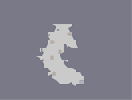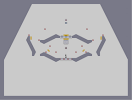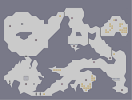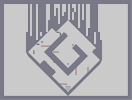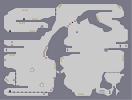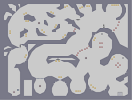Chew Herpes Cavern of Cheesecake Odjbox Cantankerous Bollocks

Pages: (0)

Sigh......
5/5

### Wooo!

Such an awesome n-art! I love it 5/5

### Script...

I have been here for longer than you. I also did not mean that these guys aren't cool, just there were some really awesome members who aren't here anymore.
and also I don't care.

### Hi ]{NO3

"the people aren't as cool IMHO. no offense guys."
Most people are going to have a hard time not taking offense to that.

As a disinterested barely-present member of NUMA, I recommend not insulting all the people whose community you're trying to join.
Woah, I remember you. Cool stuff.

### Oh heck yes.

You're alive,

and sunset is right.

the 9th doc is the way to go.

### Oh heck yes.

You're alive,

and sunset is right.

the 9th doc is the way to go.

### all doctors

are the worst doctor

5/5

.

### Neat art!

.. What a shame ..

### It's quite obvious

that 9th Doctor is best Doctor.

### Oh

yes I have... I have achieved a higher level of existence.
You obviously never saw any of the fights I got into here did you?

### "I know that I have matured somewhat in the last while"

really sounds like it.